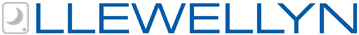X
OOPS!
VIEW CART
CONTINUE SHOPPING
XVIEW CART
CONTINUE SHOPPINGX
OOPS!
MANAGE WISHLISTS
CONTINUE SHOPPING
XCANCELLlewellyn is committed to keeping our staff and customers healthy and safe, as well as being good corporate citizens, during this time. Click to read how we are responding to the COVID-19 pandemic.Online Reference For Body, Mind & Spirit

## Calculating the Number for Your Name

 Now modern numerology, having its antecedents in the Hebrew Qabalah, is a simplification of the very complicated number system charted out by the ancient rabbis. The main difference between modern numerology and ancient gematria is the scope of symbolic attributes for the number series. The ancient Hebrew Qabalah gave deep, rich metaphors for every whole integer in the number series as far as one can count. Thus the numbers 6, 60, and 600 each has its own unique poetical number metaphor, while in modern numerology 6, 60, and 600 are each interpreted as the single digit "6." For in modern numerology only the first nine numbers are symbolically significant, the number metaphors beyond nine having been lost to time. Pythagoras Beyond the ancient Hebraic source for modern numerology, one other esoteric tradition for numbers should be mentioned: the Pythagorean school of numerical philosophy. As the Hebraic number code may have its source as far back as 1500 BCE, Pythagoras’ famous school of number philosophers was founded in Crotona around 529 BCE. Most of what we know of the Pythagorean number doctrine comes from the historical writings of Diogenes Laertius (around the third century CE). Basically, the Pythagorean doctrine views the number series as the basis from which God created the Universe. The first ten numbers are each given symbolic attributes, and ultimately any word in Greek could be calculated as a number, thus connecting word to number. Any divine name of a Greek Goddess or God could be numbered, as well as any proper name. Thus, Pythagoras’ own name by this ancient Greek numerological method was numbered as 864. By the ancient Pythagorean technique of numerology, a symbolic meaning would be given to the name Pythagoras by finding other words equal in number value to 864. But by modern numerology, to find the symbolic meaning of this name, the three digits composing the number 864 would be reduced, by addition, to a one digit number known as the digital root. Let’s try this modern method as an example. Pythagoras’ number in Greek is 864. By adding the 3 digits composing this number we get 8 + 6 + 4 = 18. Since 18 is beyond 9, we must do one more addition/reduction.7 Thus 18 become 1 + 8 = 9. Modern Numerology The letters of the English alphabet are numbered from 1 to 26. Then these numbers are reduced by adding the digits together until the result is a number from 1 to 9. By reducing the 26 letters of the English alphabet to the numbers 1 through 9 we get the following numerological chart: Number Grid for the English Alphabet 1: A, J, S 2: B, K, T 3: C, L, U 4: D, M, V 5: E, N, W 6: F, O, X 7: G, P, Y 8: H, Q, Z 9: I, R Example As an example of the use of the formula of Motives, Appearances, and Methods, let’s use the name of the founder of the Theosophical Society, Helena Petrovna Blavatsky, the most influential woman in the development of modern occultism. This name is made up of 8 vowels and 15 consonants. (Note: In modern numerology "Y" is always a consonant; the five vowels are A, E, I, O, U). The three basic calculations are as follows: 1. Motives??"The eight vowels in the name Helena Petrovna Blavatsky are E, E, A, E, O, A, A, and A, which when added together equal (E = 5) + (E = 5) + (A = 1) + (E = 5) + (O = 6) + (A = 1) + (A = 1) + (A = 1) = 25 = 2 + 5 = 7. Thus the number governing her ideals or deepest desires is 7. 2. Appearances??"The 15 consonants in Helena Petrovna Blavatsky are H, L, N, P, T, R, V, N, B, L, V, T, S, K, and Y, which when added together equal (H = 8) + (L = 3) + (N = 5) + (P = 7) + (T = 2) + (R = 9) + (V = 4) + (N = 5) + (B = 2) + (L = 3) + (V = 4) + (T = 2) + (S = 1) + (K = 2) + (Y = 7) = 64 = 6 + 4 = 10 = 1 + 0 = 1. Thus the number governing her personality is 1. 3. Methods??"The 23 letters contained in Helena Petrovna Blavatsky combine 25 (the vowels) and 64 (the consonants), totaling to 89. 89 reduces to 17, which in turn reduces to 8 (25 + 64 = 89 = 8 + 9 = 17 = 1 + 7 = 8). Thus the number governing her conduct in the world is 8. Therefore by modern numerology the name Helena Petrovna Blavatsky is generated by the number series 7:1:8.